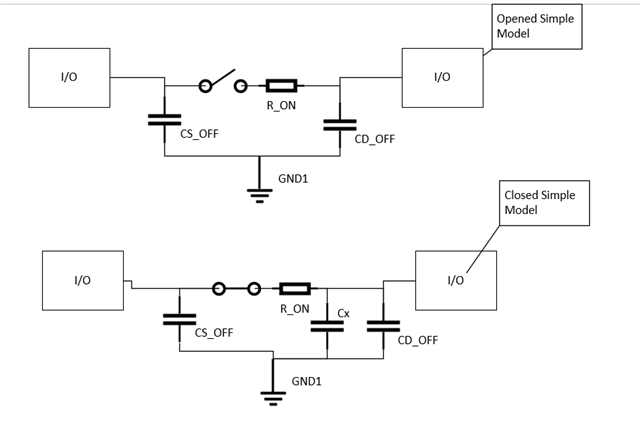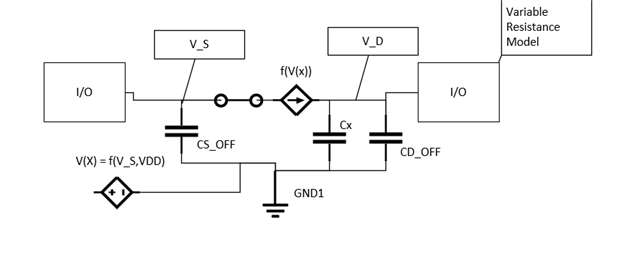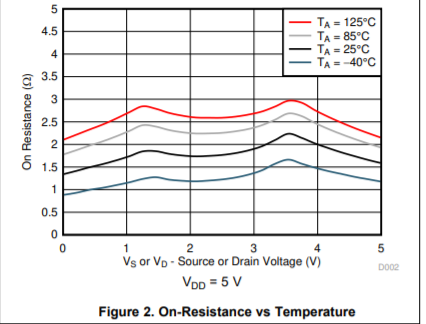If you have a related question, please click the "Ask a related question" button in the top right corner. The newly created question will be automatically linked to this question.

# TMUX1101: Tina Model

Part Number: TMUX1101
Other Parts Discussed in Thread: TMUX1119,

Hi there. Is it possible to find a Tina model of this part, in order to simulate it?

I have read someone who indicated to use TMUX1119 model, but some parameters are not the same.

Pablo.

• Hi Pablo,

You are correct there is some difference between the 1101 and 1119, so there are a couple options that we can do for the 1101 specifically.

We do have a PSpice model available for the TMUX1101 that will run in a PSpice Environment and we also have Pspice for TI which I am pretty sure is a free download of PSpice (it does  limit some imports but the TMUX1101 is there for general testing). This is the way to get the most accurate model for this device.

The next option is to use a simplified channel model which will simulate the effects of an on channel or off channel of the device. This can be broken down into a few different models - depending on what exactly wants to be modeled.

1. Simple Model - the simple model is going to be a RC circuit when the switch is closed and it will be a capacitor when the switch is open, the specs of which stated in the datasheet.For the opened switch model essentially there are the CD_Off and CS_off capacitances - at VDD = 5V -> CS_OFF= 6pF ; CD_OFF = 10pF

When the switch closes the effective capacitance of the switch C_ON = CD_OFF + CS_OFF + Cx -> since C_ON = 17pF, Cx = 1pF.

The typical On resistance is 1.8 Ohms

2. Model with Variable On Resistance

This model would be used because On resistance changes with input voltage - because how the spice circuits are setup this is actually done with a voltage controlled current source.Where f(V_S,VDD) -> is going to point to the appropriate VDD table (since on resistance is also affected by VDD), then using the V_S voltage will return with a "voltage" value that is really the resistance.

For example if we are running the 1101 at 5V VDD - a simple VX = f(V_S,5) can be made  using the R_on graphs on the datasheet:a quick example table would look like (using 25C values)

F(0,5) = 0.9

F(1,5) = 1.2

F(2,5) = 1.2

F(3,5) = 1.4

F(4,5) = 1.5

F(5,5) = 1.25

There will some approximation error but it should be relatively small - the more points added the better result you will get as well .

The current source will then convert this into a current by the formula f(V(X)) = (V_S - V_D) / Resistance (found in the first step)

3. There is one more feature that can be easily added to the model - and that is a static leakage current for the off and on conditions.

Essentially by adding a controlled current source to ground on each side for the off condition and only 1 for the on condition then using the table you can get differenent boundary conditions by using typical/ min/ and max leakage

I'd still advise to try to use the PSpice model as they will have a full featured model - but the simplified models will still give a good approximation of how the switch acts in the system when its closed or open - but it does lack the transition specs because those aren't as simple to model.

Please let me know if you have any other questions!

Best,

Parker Dodson

• Dear Parker, absolutely professional answer to my doubt. Thanks a lot. Best regards.

Pablo.